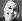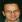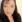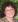# How to Put Text and a Formula into the Same Cell When Working With Excel

Knoji reviews products and up-and-coming brands we think you'll love. In certain cases, we may receive a commission from brands mentioned in our guides. Learn more.
This is a very simple, step by step guide on how to integrate text and formulas in the same Excel cell

You can get put text and a formula into the same cell of an Excel spreadsheet quite easily, which can allow you to produce very polished looking spreadsheets and reports. This is a step by step guide showing you how to make an Excel cell show text and formula results at the same time.

Speech, or quotation marks are used to let Excel know that you want what’s inside them to be treated as text, and an ampersand, the “&” symbol, is used to connect your text to the formula.

Using a simple formula

Click in cell A1 of a spread sheet and type the number 3.

Now click in cell A2 and type the number 3 in there.

Click in cell A4 and type:

=SUM(A1,A2)

Press the "Enter" key or click anywhere else on the spreadsheet. The cell A4 now shows a number “6” - the sum of 3+3 in the two cells.

The function bar (the long white strip next to the "fx" symbol) will show the formula

=SUM(A1,A2)

Showing Text in the Same Cell as a Formula

In the function bar, place your cursor after the equal symbol of your =SUM(A1,A2) formula. Type:

"The sum of 3+3 is "&

The quotation marks and the space after "is" (before the last quotation marks) are needed.

="The sum of 3+3 is "&SUM(A1,A2)

Click anywhere else on the table and cell A4 will now show: "The sum of 3+3 is 6."

Leaving a space between the last text and the second quotation mark means that there will be a gap between the result entered and the text you write.

Playing with Putting Text and a Formula into the Same Excel Cell

This technique works with whatever formula you wish to use and also works in reverse, so typing the following into the fuction bar

=SUM(A1,A2) &" is the sum of 3+3"

will present you with the words "6 is the sum of 3+3" in your cell.

You can also add text both sides at once, so if you type the formula

="The sum of 3+3 is always "&SUM(A1,B1) &" and it always will be."

You should get the following text in your cell

The sum of 3+3 is always 6 and it always will be.

Obviously you need to be a little cleverer with the maths involved before people will be impressed, but remember it’s the words and presentation that make reports feel comprehensive and professional.

This is an invaluable tool if you are automating reports, as you can place whole strings of text round your results automatically each time you create a report.Sharla Smith
0
Posted on Aug 21, 2010Rana Sinha
0
Posted on Jul 18, 2010Tanya Wallace
0
Posted on Jul 17, 2010Susan Kaul
0
Posted on Jul 13, 2010